Oracle® CEP CQL Language Reference 11g Release 1 (11.1.1) E12048-02

7 Functions: Colt Single-Row

Oracle CQL provides a variety of built-in single-row functions based on the Colt open source libraries for high performance scientific and technical computing.

7.1 Introduction to Oracle CQLBuilt-In Single-Row Colt Functions

Table 7-1 lists the built-in single-row Colt functions that Oracle CQL provides.

Table 7-1 Oracle CQL Built-in Single-Row Colt-Based Functions

Colt Package Function

cern.jet.math.Arithmetic

A set of basic polynomials, rounding, and calculus functions.

cern.jet.math.Bessel

A set of Bessel functions.

cern.jet.random.engine.RandomSeedTable

A table with good seeds for pseudo-random number generators. Each sequence in this table has a period of 10**9 numbers.

cern.jet.stat.Gamma

A set of Gamma and Beta functions.

cern.jet.stat.Probability

A set of probability distributions.

cern.colt.bitvector.QuickBitVector

A set of non polymorphic, non bounds checking, low level bit-vector functions.

cern.colt.map.HashFunctions

A set of hash functions.

 Note: Built-in function names are case sensitive and you must use them in the case shown (in lower case).

 Note: In stream input examples, lines beginning with h (such as h 3800) are heartbeat input tuples. These inform Oracle CEP that no further input will have a timestamp lesser than the heartbeat value.

beta

Syntax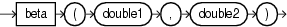Purpose

beta is based on cern.jet.stat.Gamma. It returns the beta function (see Figure 7-1) of the input arguments as a double.

Figure 7-1 cern.jet.stat.Gamma beta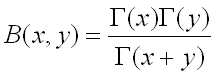This function takes the following arguments:

• double1: the x value.

• double2: the y value.

Examples

Consider the query qColt28 in Example 7-1. Given the data stream SColtFunc with schema (c1 integer, c2 double, c3 bigint) in Example 7-2, the query returns the relation in Example 7-3.

Example 7-1 beta Function Query

<query id="qColt28"><![CDATA[
select beta(c2,c2) from SColtFunc
]]></query>

Example 7-2 beta Function Stream Input

Timestamp   Tuple
10        1,0.5,8
1000        4,0.7,6
1200        3,0.89,12
2000        8,0.4,4

Example 7-3 beta Function Relation Output

Timestamp   Tuple Kind  Tuple
10:       +           3.1415927
1000:       +           1.899038
1200:       +           1.251922
2000:       +           4.226169

beta1

Syntax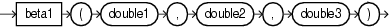Purpose

beta1 is based on cern.jet.stat.Probability. It returns the area P(x) from 0 to x under the beta density function (see Figure 7-2) as a double.

Figure 7-2 cern.jet.stat.Probability beta1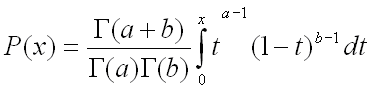This function takes the following arguments:

• double1: the alpha parameter of the beta distribution a.

• double2: the beta parameter of the beta distribution b.

• double3: the integration end point x.

Examples

Consider the query qColt35 in Example 7-4. Given the data stream SColtFunc with schema (c1 integer, c2 double, c3 bigint) in Example 7-5, the query returns the relation in Example 7-6.

Example 7-4 beta1 Function Query

<query id="qColt35"><![CDATA[
select beta1(c2,c2,c2) from SColtFunc
]]></query>

Example 7-5 beta1 Function Stream Input

Timestamp   Tuple
10        1,0.5,8
1000        4,0.7,6
1200        3,0.89,12
2000        8,0.4,4

Example 7-6 beta1 Function Relation Output

Timestamp   Tuple Kind  Tuple
10:       +           0.5
1000:       +           0.66235894
1200:       +           0.873397
2000:       +           0.44519535

betacomplemented

Syntax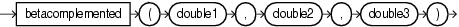Purpose

betacomplemented is based on cern.jet.stat.Probability. It returns the area under the right hand tail (from x to infinity) of the beta density function (see Figure 7-2) as a double.

This function takes the following arguments:

• double1: the alpha parameter of the beta distribution a.

• double2: the beta parameter of the beta distribution b.

• double3: the integration end point x.

Examples

Consider the query qColt37 in Example 7-7. Given the data stream SColtFunc with schema (c1 integer, c2 double, c3 bigint) in Example 7-8, the query returns the relation in Example 7-9.

Example 7-7 betacomplemented Function Query

<query id="qColt37"><![CDATA[
select betacomplemented(c2,c2,c2) from SColtFunc
]]></query>

Example 7-8 betacomplemented Function Stream Input

Timestamp   Tuple
10        1,0.5,8
1000        4,0.7,6
1200        3,0.89,12
2000        8,0.4,4

Example 7-9 betacomplemented Function Relation Output

Timestamp   Tuple Kind  Tuple
10:       +           0.5
1000:       +           0.66235894
1200:       +           0.873397
2000:       +           0.44519535

binomial

Syntax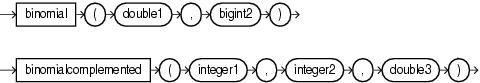Purpose

binomial is based on cern.jet.math.Arithmetic. It returns the binomial coefficient n over k (see Figure 7-3) as a double.

Figure 7-3 Definition of binomial coefficient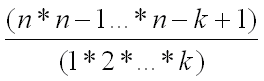This function takes the following arguments:

• double1: the n value.

• long2: the k value.

Table 7-2 lists the binomial function return values for various values of k.

Table 7-2 cern.jet.math.Arithmetic binomial Return Values

Arguments Return Value

k < 0

0

k = 0

1

k = 1

n

Any other value of k

Computed binomial coefficient as given in Figure 7-3.

Examples

Consider the query qColt6 in Example 7-10. Given the data stream SColtFunc with schema (c1 integer, c2 double, c3 long) in Example 7-11, the query returns the relation in Example 7-12.

Example 7-10 binomial Function Query

<query id="qColt6"><![CDATA[
select binomial(c2,c3) from SColtFunc
]]></query>

Example 7-11 binomial Function Stream Input

Timestamp   Tuple
10        1,0.5,8
1000        4,0.7,6
1200        3,0.89,12
2000        8,0.4,4

Example 7-12 binomial Function Relation Output

Timestamp   Tuple Kind  Tuple
10:       +           -0.013092041
1000:       +           -0.012374863
1200:       +           -0.0010145549
2000:       +           -0.0416

binomial1

Syntax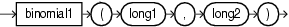Purpose

binomial1 is based on cern.jet.math.Arithmetic. It returns the binomial coefficient n over k (see Figure 7-3) as a double.

This function takes the following arguments:

• long1: the n value.

• long2: the k value.

Table 7-3 lists the BINOMIAL function return values for various values of k.

Table 7-3 cern.jet.math.Arithmetic Binomial1 Return Values

Arguments Return Value

k < 0

0

k = 0 || k = n

1

k = 1 || k = n-1

n

Any other value of k

Computed binomial coefficient as given in Figure 7-3.

Examples

Consider the query qColt7 in Example 7-13. Given the data stream SColtFunc with schema (c1 integer, c2 float, c3 long) in Example 7-14, the query returns the relation in Example 7-15.

Example 7-13 binomial1 Function Query

<query id="qColt7"><![CDATA[
select binomial1(c3,c3) from SColtFunc
]]></query>

Example 7-14 binomial1 Function Stream Input

Timestamp   Tuple
10        1,0.5,8
1000        4,0.7,6
1200        3,0.89,12
2000        8,0.4,4

Example 7-15 binomial1 Function Relation Output

Timestamp   Tuple Kind  Tuple
10:       +           1.0
1000:       +           1.0
1200:       +           1.0
2000:       +           1.0

binomial2

Syntax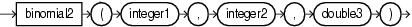Purpose

binomial2 is based on cern.jet.stat.Probability. It returns the sum of the terms 0 through k of the binomial probability density (see Figure 7-4) as a double.

Figure 7-4 cern.jet.stat.Probability binomial2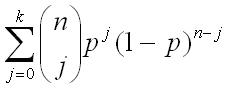This function takes the following arguments (all arguments must be positive):

• integer1: the end term k.

• integer2: the number of trials n.

• double3: the probability of success p in (0.0, 1.0)

Examples

Consider the query qColt34 in Example 7-16. Given the data stream SColtFunc with schema (c1 integer, c2 double, c3 bigint) in Example 7-17, the query returns the relation in Example 7-18.

Example 7-16 binomial2 Function Query

<query id="qColt34"><![CDATA[
select binomial2(c1,c1,c2) from SColtFunc
]]></query>

Example 7-17 binomial2 Function Stream Input

Timestamp   Tuple
10        1,0.5,8
1000        4,0.7,6
1200        3,0.89,12
2000        8,0.4,4

Example 7-18 binomial2 Function Relation Output

Timestamp   Tuple Kind  Tuple
10:       +           1.0
1000:       +           1.0
1200:       +           1.0
2000:       +           1.0

binomialcomplemented

Syntax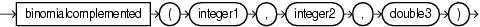Purpose

binomialcomplemented is based on cern.jet.stat.Probability. It returns the sum of the terms k+1 through n of the binomial probability density (see Figure 7-5) as a double.

Figure 7-5 cern.jet.stat.Probability binomialcomplemented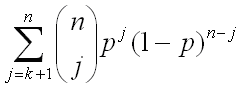This function takes the following arguments (all arguments must be positive):

• integer1: the end term k.

• integer2: the number of trials n.

• double3: the probability of success p in (0.0, 1.0)

Examples

Consider the query qColt38 in Example 7-19. Given the data stream SColtFunc with schema (integer, c2 double, c3 bigint) in Example 7-20, the query returns the relation in Example 7-21.

Example 7-19 binomialcomplemented Function Query

<query id="qColt38"><![CDATA[
select binomialcomplemented(c1,c1,c2) from SColtFunc
]]></query>

Example 7-20 binomialcomplemented Function Stream Input

Timestamp   Tuple
10        1,0.5,8
1000        4,0.7,6
1200        3,0.89,12
2000        8,0.4,4

Example 7-21 binomialcomplemented Function Relation Output

Timestamp   Tuple Kind  Tuple
10:       +           0.0
1000:       +           0.0
1200:       +           0.0
2000:       +           0.0

Syntax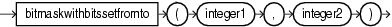Purpose

bitmaskwithbitssetfromto is based on cern.colt.bitvector.QuickBitVector. It returns a 64-bit wide bit mask as a long with bits in the specified range set to 1 and all other bits set to 0.

This function takes the following arguments:

• integer1: the from value; index of the start bit (inclusive).

• integer2: the to value; index of the end bit (inclusive).

Precondition (not checked): to - from + 1 >= 0 && to - from + 1 <= 64.

If to - from + 1 = 0 then returns a bit mask with all bits set to 0.

Examples

Consider the query qColt53 in Example 7-22. Given the data stream SColtFunc with schema (c1 integer, c2 float, c3 bigint) in Example 7-23, the query returns the relation in Example 7-24.

<query id="qColt53"><![CDATA[
]]></query>

Example 7-23 bitmaskwithbitssetfromto Function Stream Input

Timestamp   Tuple
10        1,0.5,8
1000        4,0.7,6
1200        3,0.89,12
2000        8,0.4,4

Example 7-24 bitmaskwithbitssetfromto Function Relation Output

Timestamp   Tuple Kind  Tuple
10:       +           2
1000:       +           16
1200:       +           8
2000:       +           256

ceil

Syntax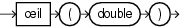Purpose

ceil is based on cern.jet.math.Arithmetic. It returns the smallest long greater than or equal to its double argument.

This method is safer than using (float) java.lang.Math.ceil(long) because of possible rounding error.

Examples

Consider the query qColt1 in Example 7-25. Given the data stream SColtFunc with schema (c1 integer, c2 double, c3 bigint) in Example 7-26, the query returns the relation in Example 7-27.

Example 7-25 ceil Function Query

<query id="qColt1"><![CDATA[
select ceil(c2) from SColtFunc
]]></query>

Example 7-26 ceil Function Stream Input

Timestamp   Tuple
10        1,0.5,8
1000        4,0.7,6
1200        3,0.89,12
2000        8,0.4,4

Example 7-27 ceil Function Relation Output

Timestamp   Tuple Kind  Tuple
10:       +           1
1000:       +           1
1200:       +           1
2000:       +           1

chisquare

Syntax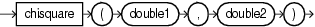Purpose

chisquare is based on cern.jet.stat.Probability. It returns the area under the left hand tail (from 0 to x) of the Chi square probability density function with v degrees of freedom (see Figure 7-6) as a double.

Figure 7-6 cern.jet.stat.Probability chisquare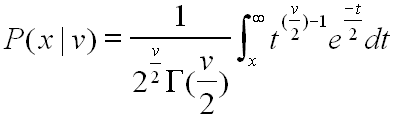This function takes the following arguments (all arguments must be positive):

• double1: the degrees of freedom v.

• double2: the integration end point x.

Examples

Consider the query qColt39 in Example 7-28. Given the data stream SColtFunc with schema (c1 integer, c2 double, c3 bigint) in Example 7-29, the query returns the relation in Example 7-30.

Example 7-28 chisquare Function Query

<query id="qColt39"><![CDATA[
select chisquare(c2,c2) from SColtFunc
]]></query>

Example 7-29 chisquare Function Stream Input

Timestamp   Tuple
10        1,0.5,8
1000        4,0.7,6
1200        3,0.89,12
2000        8,0.4,4

Example 7-30 chisquare Function Relation Output

Timestamp   Tuple Kind  Tuple
10:       +           0.0
1000:       +           0.0
1200:       +           0.0
2000:       +           0.0

chisquarecomplemented

Syntax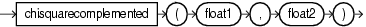Purpose

chisquarecomplemented is based on cern.jet.stat.Probability. It returns the area under the right hand tail (from x to infinity) of the Chi square probability density function with v degrees of freedom (see Figure 7-6) as a double.

This function takes the following arguments (all arguments must be positive):

• double1: the degrees of freedom v.

• double2: the integration end point x.

Examples

Consider the query qColt40 in Example 7-31. Given the data stream SColtFunc with schema (c1 integer, c2 double, c3 bigint) in Example 7-32, the query returns the relation in Example 7-33.

Example 7-31 chisquarecomplemented Function Query

<query id="qColt40"><![CDATA[
select chisquarecomplemented(c2,c2) from SColtFunc
]]></query>

Example 7-32 chisquarecomplemented Function Stream Input

Timestamp   Tuple
10        1,0.5,8
1000        4,0.7,6
1200        3,0.89,12
2000        8,0.4,4

Example 7-33 chisquarecomplemented Function Relation Output

Timestamp   Tuple Kind  Tuple
10:       +           0.0
1000:       +           0.0
1200:       +           0.0
2000:       +           0.0

errorfunction

Syntax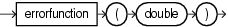Purpose

errorfunction is based on cern.jet.stat.Probability. It returns the error function of the normal distribution of the double argument as a double, using the integral that Figure 7-7 shows.

Figure 7-7 cern.jet.stat.Probability errorfunction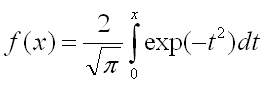Examples

Consider the query qColt41 in Example 7-34. Given the data stream SColtFunc with schema (c1 integer, c2 double, c3 bigint) in Example 7-35, the query returns the relation in Example 7-36.

Example 7-34 errorfunction Function Query

<query id="qColt41"><![CDATA[
select errorfunction(c2) from SColtFunc
]]></query>

Example 7-35 errorfunction Function Stream Input

Timestamp   Tuple
10        1,0.5,8
1000        4,0.7,6
1200        3,0.89,12
2000        8,0.4,4

Example 7-36 errorfunction Function Relation Output

Timestamp   Tuple Kind  Tuple
10:       +           0.5204999
1000:       +           0.6778012
1200:       +           0.79184324
2000:       +           0.42839235

errorfunctioncomplemented

Syntax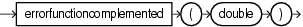Purpose

errorfunctioncomplemented is based on cern.jet.stat.Probability. It returns the complementary error function of the normal distribution of the double argument as a double, using the integral that Figure 7-8 shows.

Figure 7-8 cern.jet.stat.Probability errorfunctioncompelemented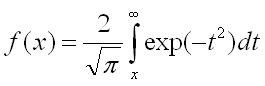Examples

Consider the query qColt42 in Example 7-37. Given the data stream SColtFunc with schema (c1 integer, c2 double, c3 bigint) in Example 7-38, the query returns the relation in Example 7-39.

Example 7-37 errorfunctioncomplemented Function Query

<query id="qColt42"><![CDATA[
select errorfunctioncomplemented(c2) from SColtFunc
]]></query>

Example 7-38 errorfunctioncomplemented Function Stream Input

Timestamp   Tuple
10        1,0.5,8
1000        4,0.7,6
1200        3,0.89,12
2000        8,0.4,4

Example 7-39 errorfunctioncomplemented Function Relation Output

Timestamp   Tuple Kind  Tuple
10:       +           0.47950011
1000:       +           0.3221988
1200:       +           0.20815676
2000:       +           0.57160765

factorial

Syntax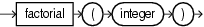Purpose

factorial is based on cern.jet.math.Arithmetic. It returns the factorial of the positive integer argument as a double.

Examples

Consider the query qColt8 in Example 7-40. Given the data stream SColtFunc with schema (c1 integer, c2 float, c3 bigint) in Example 7-41, the query returns the relation in Example 7-42.

Example 7-40 factorial Function Query

<query id="qColt8"><![CDATA[
select factorial(c1) from SColtFunc
]]></query>

Example 7-41 factorial Function Stream Input

Timestamp   Tuple
10        1,0.5,8
1000        4,0.7,6
1200        3,0.89,12
2000        8,0.4,4

Example 7-42 factorial Function Relation Output

Timestamp   Tuple Kind  Tuple
10:       +               1.0
1000:       +              24.0
1200:       +               6.0
2000:       +           40320.0

floor

Syntax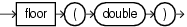Purpose

floor is based on cern.jet.math.Arithmetic. It returns the largest long value less than or equal to the double argument.

This method is safer than using (double) java.lang.Math.floor(double) because of possible rounding error.

Examples

Consider the query qColt2 in Example 7-43. Given the data stream SColtFunc with schema (c1 integer, c2 double, c3 bigint) in Example 7-44, the query returns the relation in Example 7-45.

Example 7-43 floor Function Query

<query id="qColt2"><![CDATA[
select floor(c2) from SColtFunc
]]></query>

Example 7-44 floor Function Stream Input

Timestamp   Tuple
10        1,0.5,8
1000        4,0.7,6
1200        3,0.89,12
2000        8,0.4,4

Example 7-45 floor Function Relation Output

Timestamp   Tuple Kind  Tuple
10:       +           0
1000:       +           0
1200:       +           0
2000:       +           0

gamma

Syntax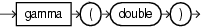Purpose

gamma is based on cern.jet.stat.Gamma. It returns the Gamma function of the double argument as a double.

Examples

Consider the query qColt29 in Example 7-46. Given the data stream SColtFunc with schema (c1 integer, c2 double, c3 bigint) in Example 7-47, the query returns the relation in Example 7-48.

Example 7-46 gamma Function Query

<query id="qColt29"><![CDATA[
select gamma(c2) from SColtFunc
]]></query>

Example 7-47 gamma Function Stream Input

Timestamp   Tuple
10        1,0.5,8
1000        4,0.7,6
1200        3,0.89,12
2000        8,0.4,4

Example 7-48 gamma Function Relation Output

Timestamp   Tuple Kind  Tuple
10:       +           1.7724539
1000:       +           1.2980554
1200:       +           1.0768307
2000:       +           2.2181594

gamma1

Syntax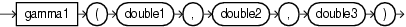Purpose

gamma1 is based on cern.jet.stat.Probability. It returns the integral from zero to x of the gamma probability density function (see Figure 7-9) as a double.

Figure 7-9 cern.jet.stat.Probability gamma1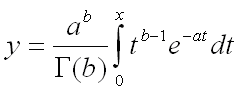This function takes the following arguments:

• double1: the gamma distribution alpha value a

• double2: the gamma distribution beta or lambda value b

• double3: the integration end point x

Examples

Consider the query qColt36 in Example 7-49. Given the data stream SColtFunc with schema (c1 integer, c2 double, c3 bigint) in Example 7-50, the query returns the relation in Example 7-51.

Example 7-49 gamma1 Function Query

<query id="qColt36"><![CDATA[
select gamma1(c2,c2,c2) from SColtFunc
]]></query>

Example 7-50 gamma1 Function Stream Input

Timestamp   Tuple
10        1,0.5,8
1000        4,0.7,6
1200        3,0.89,12
2000        8,0.4,4

Example 7-51 gamma1 Function Relation Output

Timestamp   Tuple Kind  Tuple
10:       +           0.5204999
1000:       +           0.55171627
1200:       +           0.59975785
2000:       +           0.51785487

gammacomplemented

Syntax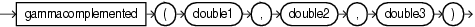Purpose

gammacomplemented is based on cern.jet.stat.Probability. It returns the integral from x to infinity of the gamma probability density function (see Figure 7-10) as a double.

Figure 7-10 cern.jet.stat.Probability gammacomplemented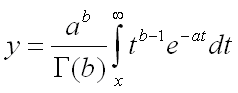This function takes the following arguments:

• double1: the gamma distribution alpha value a

• double2: the gamma distribution beta or lambda value b

• double3: the integration end point x

Examples

Consider the query qColt43 in Example 7-52. Given the data stream SColtFunc with schema (c1 integer, c2 double, c3 bigint) in Example 7-53, the query returns the relation in Example 7-54.

Example 7-52 gammacomplemented Function Query

<query id="qColt43"><![CDATA[
select gammacomplemented(c2,c2,c2) from SColtFunc
]]></query>

Example 7-53 gammacomplemented Function Stream Input

Timestamp   Tuple
10        1,0.5,8
1000        4,0.7,6
1200        3,0.89,12
2000        8,0.4,4

Example 7-54 gammacomplemented Function Relation Output

Timestamp   Tuple Kind  Tuple
10:       +           0.47950011
1000:       +           0.44828376
1200:       +           0.40024218
2000:       +           0.48214513

getseedatrowcolumn

Syntax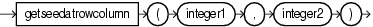Purpose

getseedatrowcolumn is based on cern.jet.random.engine.RandomSeedTable. It returns a deterministic seed as an integer from a (seemingly gigantic) matrix of predefined seeds.

This function takes the following arguments:

• integer1: the row value; should (but need not) be in [0,Integer.MAX_VALUE].

• integer2: the column value; should (but need not) be in [0,1].

Examples

Consider the query qColt27 in Example 7-55. Given the data stream SColtFunc with schema (c1 integer, c2 double, c3 bigint) in Example 7-56, the query returns the relation in Example 7-57.

Example 7-55 getseedatrowcolumn Function Query

<query id="qColt27"><![CDATA[
select getseedatrowcolumn(c1,c1) from SColtFunc
]]></query>

Example 7-56 getseedatrowcolumn Function Stream Input

Timestamp   Tuple
10        1,0.5,8
1000        4,0.7,6
1200        3,0.89,12
2000        8,0.4,4

Example 7-57 getseedatrowcolumn Function Relation Output

Timestamp   Tuple Kind  Tuple
10:       +           253987020
1000:       +           1289741558
1200:       +           417696270
2000:       +           350557787

hash

Syntax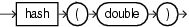Purpose

hash is based on cern.colt.map.HashFunctions. It returns an integer hashcode for the specified double value.

Examples

Consider the query qColt56 in Example 7-58. Given the data stream SColtFunc with schema (c1 integer, c2 double, c3 bigint) in Example 7-59, the query returns the relation in Example 7-60.

Example 7-58 hash Function Query

<query id="qColt56"><![CDATA[
select hash(c2) from SColtFunc
]]></query>

Example 7-59 hash Function Stream Input

Timestamp   Tuple
10        1,0.5,8
1000        4,0.7,6
1200        3,0.89,12
2000        8,0.4,4

Example 7-60 hash Function Relation Output

Timestamp   Tuple Kind  Tuple
10:       +            1071644672
1000:       +            1608935014
1200:       +            2146204385
2000:       +           -1613129319

hash1

Syntax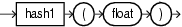Purpose

hash1 is based on cern.colt.map.HashFunctions. It returns an integer hashcode for the specified float value.

Examples

Consider the query qColt57 in Example 7-61. Given the data stream SColtFunc with schema (c1 integer, c2 double, c3 bigint) in Example 7-62, the query returns the relation in Example 7-63.

Example 7-61 hash1 Function Query

<query id="qColt57"><![CDATA[
select hash1(c2) from SColtFunc
]]></query>

Example 7-62 hash1 Function Stream Input

Timestamp   Tuple
10        1,0.5,8
1000        4,0.7,6
1200        3,0.89,12
2000        8,0.4,4

Example 7-63 hash1 Function Relation Output

Timestamp   Tuple Kind  Tuple
10:       +           1302214522
1000:       +           1306362078
1200:       +           1309462552
2000:       +           1300047248

hash2

Syntax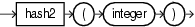Purpose

hash2 is based on cern.colt.map.HashFunctions. It returns an integer hashcode for the specified integer value.

Examples

Consider the query qColt58 in Example 7-64. Given the data stream SColtFunc with schema (c1 integer, c2 double, c3 bigint) in Example 7-65, the query returns the relation in Example 7-66.

Example 7-64 hash2 Function Query

<query id="qColt58"><![CDATA[
select hash2(c1) from SColtFunc
]]></query>

Example 7-65 hash2 Function Stream Input

Timestamp   Tuple
10        1,0.5,8
1000        4,0.7,6
1200        3,0.89,12
2000        8,0.4,4

Example 7-66 hash2 Function Relation Output

Timestamp   Tuple Kind  Tuple
10:       +           1
1000:       +           4
1200:       +           3
2000:       +           8

hash3

Syntax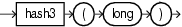Purpose

hash3 is based on cern.colt.map.HashFunctions. It returns an integer hashcode for the specified long value.

Examples

Consider the query qColt59 in Example 7-67. Given the data stream SColtFunc with schema (c1 integer, c2 double, c3 bigint) in Example 7-68, the query returns the relation in Example 7-69.

Example 7-67 hash3 Function Query

<query id="qColt59"><![CDATA[
select hash3(c3) from SColtFunc
]]></query>

Example 7-68 hash3 Function Stream Input

Timestamp   Tuple
10        1,0.5,8
1000        4,0.7,6
1200        3,0.89,12
2000        8,0.4,4

Example 7-69 hash3 Function Relation Output

Timestamp   Tuple Kind  Tuple
10:       +           8
1000:       +           6
1200:       +           12
2000:       +           4

i0

Syntax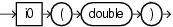Purpose

i0 is based on cern.jet.math.Bessel. It returns the modified Bessel function of order 0 of the double argument as a double.

The function is defined as i0(x) = j0(ix).

The range is partitioned into the two intervals [0,8] and (8,infinity).

Examples

Consider the query qColt12 in Example 7-70. Given the data stream SColtFunc with schema (c1 integer, c2 double, c3 bigint) in Example 7-71, the query returns the relation in Example 7-72.

Example 7-70 i0 Function Query

<query id="qColt12"><![CDATA[
select i0(c2) from SColtFunc
]]></query>

Example 7-71 i0 Function Stream Input

Timestamp   Tuple
10        1,0.5,8
1000        4,0.7,6
1200        3,0.89,12
2000        8,0.4,4

Example 7-72 i0 Function Relation Output

Timestamp   Tuple Kind  Tuple
10:       +           1.0634834
1000:       +           1.126303
1200:       +           1.2080469
2000:       +           1.0404018

i0e

SyntaxPurpose

i0e is based on cern.jet.math.Bessel. It returns the exponentially scaled modified Bessel function of order 0 of the double argument as a double.

The function is defined as: i0e(x) = exp(-|x|) j0(ix).

Examples

Consider the query qColt13 in Example 7-73. Given the data stream SColtFunc with schema (c1 integer, c2 double, c3 bigint) in Example 7-74, the query returns the relation in Example 7-75.

Example 7-73 i0e Function Query

<query id="qColt13"><![CDATA[
select i0e(c2) from SColtFunc
]]></query>

Example 7-74 i0e Function Stream Input

Timestamp   Tuple
10        1,0.5,8
1000        4,0.7,6
1200        3,0.89,12
2000        8,0.4,4

Example 7-75 i0e Function Relation Output

Timestamp   Tuple Kind  Tuple
10:       +           0.64503527
1000:       +           0.55930555
1200:       +           0.4960914
2000:       +           0.6974022

i1

Syntax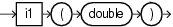Purpose

i1 is based on cern.jet.math.Bessel. It returns the modified Bessel function of order 1 of the double argument as a double.

The function is defined as: i1(x) = -i j1(ix).

The range is partitioned into the two intervals [0,8] and (8,infinity). Chebyshev polynomial expansions are employed in each interval.

Examples

Consider the query qColt14 in Example 7-76. Given the data stream SColtFunc with schema (c1 integer, c2 double, c3 bigint) in Example 7-77, the query returns the relation in Example 7-78.

Example 7-76 i1 Function Query

<query id="qColt14"><![CDATA[
select i1(c2) from SColtFunc
]]></query>

Example 7-77 i1 Function Stream Input

Timestamp   Tuple
10        1,0.5,8
1000        4,0.7,6
1200        3,0.89,12
2000        8,0.4,4

Example 7-78 i1 Function Relation Output

Timestamp   Tuple Kind  Tuple
10:       +           0.2578943
1000:       +           0.37187967
1200:       +           0.49053898
2000:       +           0.20402676

i1e

Syntax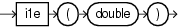Purpose

i1e is based on cern.jet.math.Bessel. It returns the exponentially scaled modified Bessel function of order 1 of the double argument as a double.

The function is defined as i1(x) = -i exp(-|x|) j1(ix).

Examples

Consider the query qColt15 in Example 7-79. Given the data stream SColtFunc with schema (c1 integer, c2 double, c3 bigint) in Example 7-80, the query returns the relation in Example 7-81.

Example 7-79 i1e Function Query

<query id="qColt15"><![CDATA[
select i1e(c2) from SColtFunc
]]></query>

Example 7-80 i1e Function Stream Input

Timestamp   Tuple
10        1,0.5,8
1000        4,0.7,6
1200        3,0.89,12
2000        8,0.4,4

Example 7-81 i1e Function Relation Output

Timestamp   Tuple Kind  Tuple
10:       +           0.1564208
1000:       +           0.18466999
1200:       +           0.20144266
2000:       +           0.13676323

incompletebeta

Syntax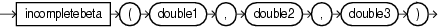Purpose

incompletebeta is based on cern.jet.stat.Gamma. It returns the Incomplete Beta Function evaluated from zero to x as a double.

This function takes the following arguments:

• double1: the beta distribution alpha value a

• double2: the beta distribution beta value b

• double3: the integration end point x

Examples

Consider the query qColt30 in Example 7-82. Given the data stream SColtFunc with schema (c1 integer, c2 double, c3 bigint) in Example 7-83, the query returns the relation in Example 7-84.

Example 7-82 incompletebeta Function Query

<query id="qColt30"><![CDATA[
select incompletebeta(c2,c2,c2) from SColtFunc
]]></query>

Example 7-83 incompletebeta Function Stream Input

Timestamp   Tuple
10        1,0.5,8
1000        4,0.7,6
1200        3,0.89,12
2000        8,0.4,4

Example 7-84 incompletebeta Function Relation Output

Timestamp   Tuple Kind  Tuple
10:       +           0.5
1000:       +           0.66235894
1200:       +           0.873397
2000:       +           0.44519535

incompletegamma

Syntax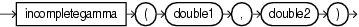Purpose

incompletegamma is based on cern.jet.stat.Gamma. It returns the Incomplete Gamma function of the arguments as a double.

This function takes the following arguments:

• double1: the gamma distribution alpha value a.

• double2: the integration end point x.

Examples

Consider the query qColt31 in Example 7-85. Given the data stream SColtFunc with schema (c1 integer, c2 double, c3 bigint) in Example 7-86, the query returns the relation in Example 7-87.

Example 7-85 incompletegamma Function Query

<query id="qColt31"><![CDATA[
select incompletegamma(c2,c2) from SColtFunc
]]></query>

Example 7-86 incompletegamma Function Stream Input

Timestamp   Tuple
10        1,0.5,8
1000        4,0.7,6
1200        3,0.89,12
2000        8,0.4,4

Example 7-87 incompletegamma Function Relation Output

Timestamp   Tuple Kind  Tuple
10:       +           0.6826895
1000:       +           0.6565891
1200:       +           0.6397422
2000:       +           0.7014413

incompletegammacomplement

Syntax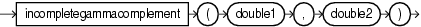Purpose

incompletegammacomplement is based on cern.jet.stat.Gamma. It returns the Complemented Incomplete Gamma function of the arguments as a double.

This function takes the following arguments:

• double1: the gamma distribution alpha value a.

• double2: the integration start point x.

Examples

Consider the query qColt32 in Example 7-88. Given the data stream SColtFunc with schema (c1 integer, c2 double, c3 bigint) in Example 7-89, the query returns the relation in Example 7-90.

Example 7-88 incompletegammacomplement Function Query

<query id="qColt32"><![CDATA[
select incompletegammacomplement(c2,c2) from SColtFunc
]]></query>

Example 7-89 incompletegammacomplement Function Stream Input

Timestamp   Tuple
10        1,0.5,8
1000        4,0.7,6
1200        3,0.89,12
2000        8,0.4,4

Example 7-90 incompletegammacomplement Function Relation Output

Timestamp   Tuple Kind  Tuple
10:       +           0.3173105
1000:       +           0.34341094
1200:       +           0.3602578
2000:       +           0.29855874

j0

Syntax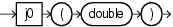Purpose

j0 is based on cern.jet.math.Bessel. It returns the Bessel function of the first kind of order 0 of the double argument as a double.

Examples

Consider the query qColt16 in Example 7-91. Given the data stream SColtFunc with schema (c1 integer, c2 double, c3 bigint) in Example 7-92, the query returns the relation in Example 7-93.

Example 7-91 j0 Function Query

<query id="qColt16"><![CDATA[
select j0(c2) from SColtFunc
]]></query>

Example 7-92 j0 Function Stream Input

Timestamp   Tuple
10        1,0.5,8
1000        4,0.7,6
1200        3,0.89,12
2000        8,0.4,4

Example 7-93 j0 Function Relation Output

Timestamp   Tuple Kind  Tuple
10:       +           0.9384698
1000:       +           0.8812009
1200:       +           0.8115654
2000:       +           0.9603982

j1

Syntax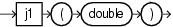Purpose

j1 is based on cern.jet.math.Bessel. It returns the Bessel function of the first kind of order 1 of the double argument as a double.

Examples

Consider the query qColt17 in Example 7-94. Given the data stream SColtFunc with schema (c1 integer, c2 double, c3 bigint) in Example 7-95, the query returns the relation in Example 7-96.

Example 7-94 j1 Function Query

<query id="qColt17"><![CDATA[
select j1(c2) from SColtFunc
]]></query>

Example 7-95 j1 Function Stream Input

Timestamp   Tuple
10        1,0.5,8
1000        4,0.7,6
1200        3,0.89,12
2000        8,0.4,4

Example 7-96 j1 Function Relation Output

Timestamp   Tuple Kind  Tuple
10:       +           0.24226846
1000:       +           0.32899573
1200:       +           0.40236986
2000:       +           0.19602658

jn

Syntax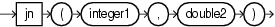Purpose

jn is based on cern.jet.math.Bessel. It returns the Bessel function of the first kind of order n of the argument as a double.

This function takes the following arguments:

• integer1: the order of the Bessel function n.

• double2: the value to compute the bessel function of x.

Examples

Consider the query qColt18 in Example 7-97. Given the data stream SColtFunc with schema (c1 integer, c2 double, c3 bigint) in Example 7-98, the query returns the relation in Example 7-99.

Example 7-97 jn Function Query

<query id="qColt18"><![CDATA[
select jn(c1,c2) from SColtFunc
]]></query>

Example 7-98 jn Function Stream Input

Timestamp   Tuple
10        1,0.5,8
1000        4,0.7,6
1200        3,0.89,12
2000        8,0.4,4

Example 7-99 jn Function Relation Output

Timestamp   Tuple Kind  Tuple
10:       +           0.24226846
1000:       +           6.1009696E-4
1200:       +           0.0139740035
2000:       +           6.321045E-11

k0

Syntax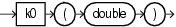Purpose

k0 is based on cern.jet.math.Bessel. It returns the modified Bessel function of the third kind of order 0 of the double argument as a double.

The range is partitioned into the two intervals [0,8] and (8, infinity). Chebyshev polynomial expansions are employed in each interval.

Examples

Consider the query qColt19 in Example 7-100. Given the data stream SColtFunc with schema (c1 integer, c2 double, c3 bigint) in Example 7-101, the query returns the relation in Example 7-102.

Example 7-100 k0 Function Query

<query id="qColt19"><![CDATA[
select k0(c2) from SColtFunc
]]></query>

Example 7-101 k0 Function Stream Input

Timestamp   Tuple
10        1,0.5,8
1000        4,0.7,6
1200        3,0.89,12
2000        8,0.4,4

Example 7-102 k0 Function Relation Output

Timestamp   Tuple Kind  Tuple
10:       +           0.92441905
1000:       +           0.6605199
1200:       +           0.49396032
2000:       +           1.1145291

k0e

Syntax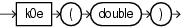Purpose

k0e is based on cern.jet.math.Bessel. It returns the exponentially scaled modified Bessel function of the third kind of order 0 of the double argument as a double.

Examples

Consider the query qColt20 in Example 7-103. Given the data stream SColtFunc with schema (c1 integer, c2 double, c3 bigint) in Example 7-104, the query returns the relation in Example 7-105.

Example 7-103 k0e Function Query

<query id="qColt20"><![CDATA[
select k0e(c2) from SColtFunc
]]></query>

Example 7-104 k0e Function Stream Input

Timestamp   Tuple
10        1,0.5,8
1000        4,0.7,6
1200        3,0.89,12
2000        8,0.4,4

Example 7-105 k0e Function Relation Output

Timestamp   Tuple Kind  Tuple
10:       +           1.5241094
1000:       +           1.3301237
1200:       +           1.2028574
2000:       +           1.662682

k1

Syntax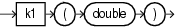Purpose

k1 is based on cern.jet.math.Bessel. It returns the modified Bessel function of the third kind of order 1 of the double argument as a double.

The range is partitioned into the two intervals [0,2] and (2, infinity). Chebyshev polynomial expansions are employed in each interval.

Examples

Consider the query qColt21 in Example 7-106. Given the data stream SColtFunc with schema (c1 integer, c2 double, c3 bigint) in Example 7-107, the query returns the relation in Example 7-108.

Example 7-106 k1 Function Query

<query id="qColt21"><![CDATA[
select k1(c2) from SColtFunc
]]></query>

Example 7-107 k1 Function Stream Input

Timestamp   Tuple
10        1,0.5,8
1000        4,0.7,6
1200        3,0.89,12
2000        8,0.4,4

Example 7-108 k1 Function Relation Output

Timestamp   Tuple Kind  Tuple
10:       +           1.6564411
1000:       +           1.0502836
1200:       +           0.7295154
2000:       +           2.1843543

k1e

SyntaxPurpose

k1e is based on cern.jet.math.Bessel. It returns the exponentially scaled modified Bessel function of the third kind of order 1 of the double argument as a double.

The function is defined as: k1e(x) = exp(x) * k1(x).

Examples

Consider the query qColt22 in Example 7-109. Given the data stream SColtFunc with schema (c1 integer, c2 double, c3 bigint) in Example 7-110, the query returns the relation in Example 7-111.

Example 7-109 k1e Function Query

<query id="qColt22"><![CDATA[
select k1e(c2) from SColtFunc
]]></query>

Example 7-110 k1e Function Stream Input

Timestamp   Tuple
10        1,0.5,8
1000        4,0.7,6
1200        3,0.89,12
2000        8,0.4,4

Example 7-111 k1e Function Relation Output

Timestamp   Tuple Kind  Tuple
10:       +           2.7310097
1000:       +           2.1150115
1200:       +           1.7764645
2000:       +           3.258674

kn

Syntax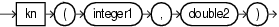Purpose

kn is based on cern.jet.math.Bessel. It returns the modified Bessel function of the third kind of order n of the argument as a double.

This function takes the following arguments:

• integer1: the n value order of the Bessel function.

• double2: the x value to compute the bessel function of.

The range is partitioned into the two intervals [0,9.55] and (9.55, infinity). An ascending power series is used in the low range, and an asymptotic expansion in the high range.

Examples

Consider the query qColt23 in Example 7-112. Given the data stream SColtFunc with schema (c1 integer, c2 double, c3 bigint) in Example 7-113, the query returns the relation in Example 7-114.

Example 7-112 kn Function Query

<query id="qColt23"><![CDATA[
select kn(c1,c2) from SColtFunc
]]></query>

Example 7-113 kn Function Stream Input

Timestamp   Tuple
10        1,0.5,8
1000        4,0.7,6
1200        3,0.89,12
2000        8,0.4,4

Example 7-114 kn Function Relation Output

Timestamp   Tuple Kind  Tuple
10:       +             1.6564411
1000:       +           191.99422
1200:       +            10.317473
2000:       +             9.7876858E8

leastsignificantbit

Syntax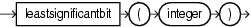Purpose

leastsignificantbit is based on cern.colt.bitvector.QuickBitVector. It returns the index (as an integer) of the least significant bit in state true of the integer argument. Returns 32 if no bit is in state true.

Examples

Consider the query qColt54 in Example 7-115. Given the data stream SColtFunc with schema (c1 integer, c2 double, c3 bigint) in Example 7-116, the query returns the relation in Example 7-117.

Example 7-115 leastsignificantbit Function Query

<query id="qColt54"><![CDATA[
select leastsignificantbit(c1) from SColtFunc
]]></query>

Example 7-116 leastsignificantbit Function Stream Input

Timestamp   Tuple
10        1,0.5,8
1000        4,0.7,6
1200        3,0.89,12
2000        8,0.4,4

Example 7-117 leastsignificantbit Function Relation Output

Timestamp   Tuple Kind  Tuple
10:       +           0
1000:       +           2
1200:       +           0
2000:       +           3

log

Syntax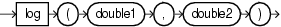Purpose

log is based on cern.jet.math.Arithmetic. It returns the computation that Figure 7-11 shows as a double.

Figure 7-11 cern.jet.math.Arithmetic log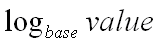This function takes the following arguments:

• double1: the base.

• double2: the value.

Examples

Consider the query qColt3 in Example 7-118. Given the data stream SColtFunc with schema (c1 integer, c2 double, c3 bigint) in Example 7-119, the query returns the relation in Example 7-120.

Example 7-118 log Function Query

<query id="qColt3"><![CDATA[
select log(c2,c2) from SColtFunc
]]></query>

Example 7-119 log Function Stream Input

Timestamp   Tuple
10        1,0.5,8
1000        4,0.7,6
1200        3,0.89,12
2000        8,0.4,4

Example 7-120 log Function Relation Output

Timestamp   Tuple Kind  Tuple
10:       +           1.0
1000:       +           1.0
1200:       +           1.0
2000:       +           1.0

log10

Syntax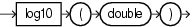Purpose

log10 is based on cern.jet.math.Arithmetic. It returns the base 10 logarithm of a double value as a double.

Examples

Consider the query qColt4 in Example 7-121. Given the data stream SColtFunc with schema (c1 integer, c2 double, c3 bigint) in Example 7-122, the query returns the relation in Example 7-123.

Example 7-121 log10 Function Query

<query id="qColt4"><![CDATA[
select log10(c2) from SColtFunc
]]></query>

Example 7-122 log10 Function Stream Input

Timestamp   Tuple
10        1,0.5,8
1000        4,0.7,6
1200        3,0.89,12
2000        8,0.4,4

Example 7-123 log10 Function Relation Output

Timestamp   Tuple Kind  Tuple
10:       +           -0.30103
1000:       +           -0.15490197
1200:       +           -0.050610002
2000:       +           -0.39794

log2

Syntax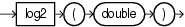Purpose

log2 is based on cern.jet.math.Arithmetic. It returns the base 2 logarithm of a double value as a double.

Examples

Consider the query qColt9 in Example 7-124. Given the data stream SColtFunc with schema (c1 integer, c2 double, c3 bigint) in Example 7-125, the query returns the relation in Example 7-126.

Example 7-124 log2 Function Query

<query id="qColt9"><![CDATA[
select log2(c2) from SColtFunc
]]></query>

Example 7-125 log2 Function Stream Input

Timestamp   Tuple
10        1,0.5,8
1000        4,0.7,6
1200        3,0.89,12
2000        8,0.4,4

Example 7-126 log2 Function Relation Output

Timestamp   Tuple Kind  Tuple
10:       +           -1.0
1000:       +           -0.5145732
1200:       +           -0.16812278
2000:       +           -1.321928

logfactorial

Syntax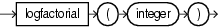Purpose

logfactorial is based on cern.jet.math.Arithmetic. It returns the natural logarithm (base e) of the factorial of its integer argument as a double

For argument values k<30, the function looks up the result in a table in O(1). For argument values k>=30, the function uses Stirlings approximation.

Examples

Consider the query qColt10 in Example 7-127. Given the data stream SColtFunc with schema (c1 integer, c2 double, c3 bigint) in Example 7-128, the query returns the relation in Example 7-129.

Example 7-127 logfactorial Function Query

<query id="qColt10"><![CDATA[
select logfactorial(c1) from SColtFunc
]]></query>

Example 7-128 logfactorial Function Stream Input

Timestamp   Tuple
10        1,0.5,8
1000        4,0.7,6
1200        3,0.89,12
2000        8,0.4,4

Example 7-129 logfactorial Function Relation Output

Timestamp   Tuple Kind  Tuple
10:       +            0.0
1000:       +            3.1780539
1200:       +            1.7917595
2000:       +           10.604603

loggamma

Syntax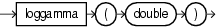Purpose

loggamma is based on cern.jet.stat.Gamma. It returns the natural logarithm (base e) of the gamma function of the double argument as a double.

Examples

Consider the query qColt33 in Example 7-130. Given the data stream SColtFunc with schema (c1 integer, c2 double, c3 bigint) in Example 7-131, the query returns the relation in Example 7-132.

Example 7-130 loggamma Function Query

<query id="qColt33"><![CDATA[
select loggamma(c2) from SColtFunc
]]></query>

Example 7-131 loggamma Function Stream Input

Timestamp   Tuple
10        1,0.5,8
1000        4,0.7,6
1200        3,0.89,12
2000        8,0.4,4

Example 7-132 loggamma Function Relation Output

Timestamp   Tuple Kind  Tuple
10:       +           0.5723649
1000:       +           0.26086727
1200:       +           0.07402218
2000:       +           0.7966778

longfactorial

Syntax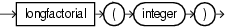Purpose

longfactorial is based on cern.jet.math.Arithmetic. It returns the factorial of its integer argument (in the range k >= 0 && k < 21) as a long.

Examples

Consider the query qColt11 in Example 7-133. Given the data stream SColtFunc with schema (c1 integer, c2 double, c3 bigint) in Example 7-134, the query returns the relation in Example 7-135.

Example 7-133 longfactorial Function Query

<query id="qColt11"><![CDATA[
select longfactorial(c1) from SColtFunc
]]></query>

Example 7-134 longfactorial Function Stream Input

Timestamp   Tuple
10        1,0.5,8
1000        4,0.7,6
1200        3,0.89,12
2000        8,0.4,4

Example 7-135 longfactorial Function Relation Output

Timestamp   Tuple Kind  Tuple
10:       +           1
1000:       +           24
1200:       +           6
2000:       +           40320

mostsignificantbit

Syntax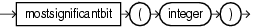Purpose

mostsignificantbit is based on cern.colt.bitvector.QuickBitVector. It returns the index (as an integer) of the most significant bit in state true of the integer argument. Returns -1 if no bit is in state true

Examples

Consider the query qColt55 in Example 7-136. Given the data stream SColtFunc with schema (c1 integer, c2 double, c3 bigint) in Example 7-137, the query returns the relation in Example 7-138.

Example 7-136 mostsignificantbit Function Query

<query id="qColt55"><![CDATA[
select mostsignificantbit(c1) from SColtFunc
]]></view>

Example 7-137 mostsignificantbit Function Stream Input

Timestamp   Tuple
10        1,0.5,8
1000        4,0.7,6
1200        3,0.89,12
2000        8,0.4,4

Example 7-138 mostsignificantbit Function Relation Output

Timestamp   Tuple Kind  Tuple
10:       +           0
1000:       +           2
1200:       +           1
2000:       +           3

negativebinomial

Syntax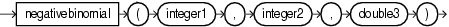Purpose

negativebinomial is based on cern.jet.stat.Probability. It returns the sum of the terms 0 through k of the Negative Binomial Distribution (see Figure 7-12) as a double.

Figure 7-12 cern.jet.stat.Probability negativebinomial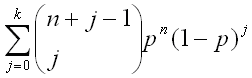This function takes the following arguments:

• integer1: the end term k.

• integer2: the number of trials n.

• double3: the probability of success p in (0.0,1.0).

Examples

Consider the query qColt44 in Example 7-139. Given the data stream SColtFunc with schema (c1 integer, c2 double, c3 bigint) in Example 7-140, the query returns the relation in Example 7-141.

Example 7-139 negativebinomial Function Query

<query id="qColt44"><![CDATA[
select negativebinomial(c1,c1,c2) from SColtFunc
]]></query>

Example 7-140 negativebinomial Function Stream Input

Timestamp   Tuple
10        1,0.5,8
1000        4,0.7,6
1200        3,0.89,12
2000        8,0.4,4

Example 7-141 negativebinomial Function Relation Output

Timestamp   Tuple Kind  Tuple
10:       +           0.75
1000:       +           0.94203234
1200:       +           0.99817264
2000:       +           0.28393665

negativebinomialcomplemented

Syntax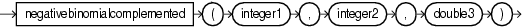Purpose

negativebinomialcomplemented is based on cern.jet.stat.Probability. It returns the sum of the terms k+1 to infinity of the Negative Binomial distribution (see Figure 7-13) as a double.

Figure 7-13 cern.jet.stat.Probability negativebinomialcomplementedThis function takes the following arguments:

• integer1: the end term k.

• integer2: the number of trials n.

• double3: the probability of success p in (0.0,1.0).

Examples

Consider the query qColt45 in Example 7-142. Given the data stream SColtFunc with schema (c1 integer, c2 double, c3 bigint) in Example 7-143, the query returns the relation in Example 7-144.

Example 7-142 negativebinomialcomplemented Function Query

<query id="qColt45"><![CDATA[
select negativebinomialcomplemented(c1,c1,c2) from SColtFunc
]]></query>

Example 7-143 negativebinomialcomplemented Function Stream Input

Timestamp   Tuple
10        1,0.5,8
1000        4,0.7,6
1200        3,0.89,12
2000        8,0.4,4

Example 7-144 negativebinomialcomplemented Function Relation Output

Timestamp   Tuple Kind  Tuple
10:       +           0.25
1000:       +           0.05796766
1200:       +           0.0018273441
2000:       +           0.7160633

normal

Syntax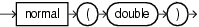Purpose

normal is based on cern.jet.stat.Probability. It returns the area under the Normal (Gaussian) probability density function, integrated from minus infinity to the double argument x (see Figure 7-14) as a double.

Figure 7-14 cern.jet.stat.Probability normal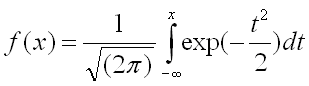Examples

Consider the query qColt46 in Example 7-145. Given the data stream SColtFunc with schema (c1 integer, c2 double, c3 bigint) in Example 7-146, the query returns the relation in Example 7-147.

Example 7-145 normal Function Query

<query id="qColt46"><![CDATA[
select normal(c2) from SColtFunc
]]></query>

Example 7-146 normal Function Stream Input

Timestamp   Tuple
10        1,0.5,8
1000        4,0.7,6
1200        3,0.89,12
2000        8,0.4,4

Example 7-147 normal Function Relation Output

Timestamp   Tuple Kind  Tuple
10:       +           0.69146246
1000:       +           0.7580363
1200:       +           0.81326705
2000:       +           0.65542173

normal1

Syntax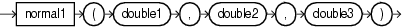Purpose

normal1 is based on cern.jet.stat.Probability. It returns the area under the Normal (Gaussian) probability density function, integrated from minus infinity to x (see Figure 7-15) as a double.

Figure 7-15 cern.jet.stat.Probability normal1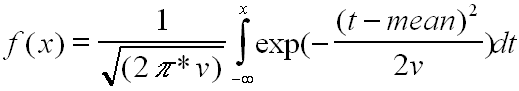This function takes the following arguments:

• double1: the normal distribution mean.

• double2: the variance of the normal distribution v.

• double3: the integration limit x.

Examples

Consider the query qColt47 in Example 7-148. Given the data stream SColtFunc with schema (c1 integer, c2 double, c3 bigint) in Example 7-149, the query returns the relation in Example 7-150.

Example 7-148 normal1 Function Query

<query id="qColt47"><![CDATA[
select normal1(c2,c2,c2) from SColtFunc
]]></query>

Example 7-149 normal1 Function Stream Input

Timestamp   Tuple
10        1,0.5,8
1000        4,0.7,6
1200        3,0.89,12
2000        8,0.4,4

Example 7-150 normal1 Function Relation Output

Timestamp   Tuple Kind  Tuple
10:       +           0.5
1000:       +           0.5
1200:       +           0.5
2000:       +           0.5

normalinverse

Syntax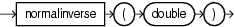Purpose

normalinverse is based on cern.jet.stat.Probability. It returns the double value, x, for which the area under the Normal (Gaussian) probability density function (integrated from minus infinity to x) equals the double argument y (assumes mean is zero and variance is one).

Examples

Consider the query qColt48 in Example 7-151. Given the data stream SColtFunc with schema (c1 integer, c2 double, c3 bigint) in Example 7-152, the query returns the relation in Example 7-153.

Example 7-151 normalinverse Function Query

<query id="qColt48"><![CDATA[
select normalinverse(c2) from SColtFunc
]]></view>

Example 7-152 normalinverse Function Stream Input

Timestamp   Tuple
10        1,0.5,8
1000        4,0.7,6
1200        3,0.89,12
2000        8,0.4,4

Example 7-153 normalinverse Function Relation Output

Timestamp   Tuple Kind  Tuple
10:       +           0.0
1000:       +           0.5244005
1200:       +           1.226528
2000:       +           0.2533471

poisson

Syntax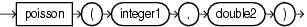Purpose

poisson is based on cern.jet.stat.Probability. It returns the sum of the first k terms of the Poisson distribution (see Figure 7-16) as a double.

Figure 7-16 cern.jet.stat.Probability poisson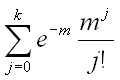This function takes the following arguments:

• integer1: the number of terms k.

• double2: the mean of the Poisson distribution m.

Examples

Consider the query qColt49 in Example 7-154. Given the data stream SColtFunc with schema (c1 integer, c2 double, c3 bigint) in Example 7-155, the query returns the relation in Example 7-156.

Example 7-154 poisson Function Query

<query id="qColt49"><![CDATA[
select poisson(c1,c2) from SColtFunc
]]></query>

Example 7-155 poisson Function Stream Input

Timestamp   Tuple
10        1,0.5,8
1000        4,0.7,6
1200        3,0.89,12
2000        8,0.4,4

Example 7-156 poisson Function Relation Output

Timestamp   Tuple Kind  Tuple
10:       +           0.909796
1000:       +           0.9992145
1200:       +           0.9870295
2000:       +           1.0

poissoncomplemented

Syntax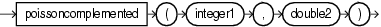Purpose

poissoncomplemented is based on cern.jet.stat.Probability. It returns the sum of the terms k+1 to Infinity of the Poisson distribution (see Figure 7-17) as a double.

Figure 7-17 cern.jet.stat.Probability poissoncomplemented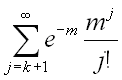This function takes the following arguments:

• integer1: the start term k.

• double2: the mean of the Poisson distribution m.

Examples

Consider the query qColt50 in Example 7-157. Given the data stream SColtFunc with schema (c1 integer, c2 double, c3 bigint) in Example 7-158, the query returns the relation in Example 7-159.

Example 7-157 poissoncomplemented Function Query

<query id="qColt50"><![CDATA[
select poissoncomplemented(c1,c2) from SColtFunc
]]></query>

Example 7-158 poissoncomplemented Function Stream Input

Timestamp   Tuple
10        1,0.5,8
1000        4,0.7,6
1200        3,0.89,12
2000        8,0.4,4

Example 7-159 poissoncomplemented Function Relation Output

Timestamp   Tuple Kind  Tuple
10:       +           0.09020401
1000:       +           7.855354E-4
1200:       +           0.012970487
2000:       +           5.043364E-10

stirlingcorrection

Syntax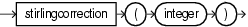Purpose

stirlingcorrection is based on cern.jet.math.Arithmetic. It returns the correction term of the Stirling approximation of the natural logarithm (base e) of the factorial of the integer argument (see Figure 7-18) as a double.

Figure 7-18 cern.jet.math.Arithmetic stirlingcorrection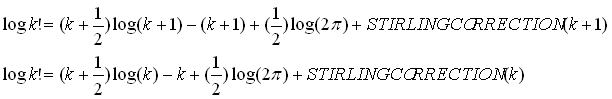Examples

Consider the query qColt5 in Example 7-160. Given the data stream SColtFunc with schema (c1 integer, c2 double, c3 bigint) in Example 7-161, the query returns the relation in Example 7-162.

Example 7-160 stirlingcorrection Function Query

<query id="qColt5"><![CDATA[
select stirlingcorrection(c1) from SColtFunc
]]></query>

Example 7-161 stirlingcorrection Function Stream Input

Timestamp   Tuple
10        1,0.5,8
1000        4,0.7,6
1200        3,0.89,12
2000        8,0.4,4

Example 7-162 stirlingcorrection Function Relation Output

Timestamp   Tuple Kind  Tuple
10:       +           0.08106147
1000:       +           0.020790672
1200:       +           0.027677925
2000:       +           0.010411265

studentt

Syntax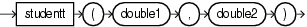Purpose

studentt is based on cern.jet.stat.Probability. It returns the integral from minus infinity to t of the Student-t distribution with k > 0 degrees of freedom (see Figure 7-19) as a double.

Figure 7-19 cern.jet.stat.Probability studentt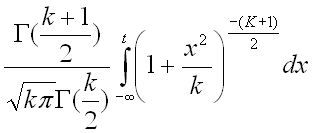This function takes the following arguments:

• double1: the degrees of freedom k.

• double2: the integration end point t.

Examples

Consider the query qColt51 in Example 7-163. Given the data stream SColtFunc with schema (c1 integer, c2 double, c3 bigint) in Example 7-164, the query returns the relation in Example 7-165.

Example 7-163 studentt Function Query

<query id="qColt51"><![CDATA[
select studentt(c2,c2) from SColtFunc
]]></query>

Example 7-164 studentt Function Stream Input

Timestamp   Tuple
10        1,0.5,8
1000        4,0.7,6
1200        3,0.89,12
2000        8,0.4,4

Example 7-165 studentt Function Relation Output

Timestamp   Tuple Kind  Tuple
10:       +           0.621341
1000:       +           0.67624015
1200:       +           0.7243568
2000:       +           0.5930112

studenttinverse

Syntax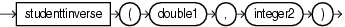Purpose

studenttinverse is based on cern.jet.stat.Probability. It returns the double value, t, for which the area under the Student-t probability density function (integrated from minus infinity to t) equals 1-alpha/2. The value returned corresponds to the usual Student t-distribution lookup table for talpha[size]. This function uses the studentt function to determine the return value iteratively.

This function takes the following arguments:

• double1: the probability alpha.

• integer2: the data set size.

Examples

Consider the query qColt52 in Example 7-166. Given the data stream SColtFunc with schema (c1 integer, c2 double, c3 bigint) in Example 7-167, the query returns the relation in Example 7-168.

Example 7-166 studenttinverse Function Query

<query id="qColt52"><![CDATA[
select studenttinverse(c2,c1) from SColtFunc
]]></query>

Example 7-167 studenttinverse Function Stream Input

Timestamp   Tuple
10        1,0.5,8
1000        4,0.7,6
1200        3,0.89,12
2000        8,0.4,4

Example 7-168 studenttinverse Function Relation Output

Timestamp   Tuple Kind  Tuple
10:       +           1.0
1000:       +           0.4141633
1200:       +           0.15038916
2000:       +           0.8888911

y0

Syntax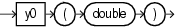Purpose

y0 is based on cern.jet.math.Bessel. It returns the Bessel function of the second kind of order 0 of the double argument as a double.

Examples

Consider the query qColt24 in Example 7-169. Given the data stream SColtFunc with schema (c1 integer, c2 double, c3 bigint) in Example 7-170, the query returns the relation in Example 7-171.

Example 7-169 y0 Function Query

<query id="qColt24"><![CDATA[
select y0(c2) from SColtFunc
]]></query>

Example 7-170 y0 Function Stream Input

Timestamp   Tuple
10        1,0.5,8
1000        4,0.7,6
1200        3,0.89,12
2000        8,0.4,4

Example 7-171 y0 Function Relation Output

Timestamp   Tuple Kind  Tuple
10:       +           -0.44451874
1000:       +           -0.19066493
1200:       +           -0.0031519707
2000:       +           -0.60602456

y1

Syntax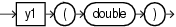Purpose

y1 is based on cern.jet.math.Bessel. It returns the Bessel function of the second kind of order 1 of the float argument as a double.

Examples

Consider the query qColt25 in Example 7-172. Given the data stream SColtFunc with schema (c1 integer, c2 double, c3 bigint) in Example 7-173, the query returns the relation in Example 7-174.

Example 7-172 y1 Function Query

<query id="qColt25"><![CDATA[
select y1(c2) from SColtFunc
]]></query>

Example 7-173 y1 Function Stream Input

Timestamp   Tuple
10        1,0.5,8
1000        4,0.7,6
1200        3,0.89,12
2000        8,0.4,4

Example 7-174 y1 Function Relation Output

Timestamp   Tuple Kind  Tuple
10:       +           -1.4714724
1000:       +           -1.1032499
1200:       +           -0.88294965
2000:       +           -1.780872

yn

Syntax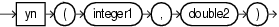Purpose

yn is based on cern.jet.math.Bessel. It returns the Bessel function of the second kind of order n of the double argument as a double.

This function takes the following arguments:

• integer1: the n value order of the Bessel function.

• double2: the x value to compute the Bessel function of.

Examples

Consider the query qColt26 in Example 7-175. Given the data stream SColtFunc with schema (c1 integer, c2 double, c3 bigint) in Example 7-176, the query returns the relation in Example 7-177.

Example 7-175 yn Function Query

<query id="qColt26"><![CDATA[
select yn(c1,c2) from SColtFunc
]]></query>

Example 7-176 yn Function Stream Input

Timestamp   Tuple
10        1,0.5,8
1000        4,0.7,6
1200        3,0.89,12
2000        8,0.4,4

Example 7-177 yn Function Relation Output

Timestamp   Tuple Kind  Tuple
10:       +             -1.4714724
1000:       +           -132.63406
1200:       +             -8.020442
2000:       +             -6.3026547E8